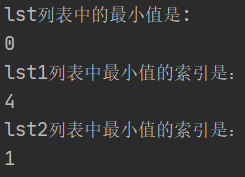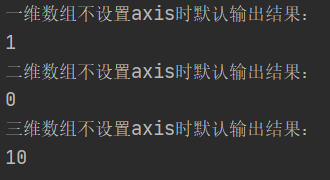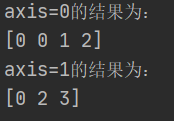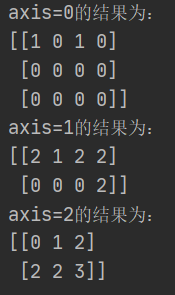# 全网最详细的numpy argmin和argmax分析（一次性理解np.argmin）

``````def argmin(a, axis=None, out=None):
"""
Returns the indices of the minimum values along an axis.

Parameters
----------
a : array_like
Input array.
axis : int, optional
By default, the index is into the flattened array, otherwise
along the specified axis.
out : array, optional
If provided, the result will be inserted into this array. It should
be of the appropriate shape and dtype.``````

1.若参数a为list类型

np.argmin(a)返回将a中所有元素全部按照原有顺序拉直摊平之后，第一个最小值在其中的位置，举例说明：

``````import numpy as np

lst1=[1,100,56,78,0]
lst2=[[100,0,5],[3,0,7],[5,0,6]]
print("lst列表中的最小值是:")
print(np.min(lst1))
# 仅有一个最小值的情况
print("lst1列表中最小值的索引是：")
print(np.argmin(lst1))
# 有多个最小值的情况
print("lst2列表中最小值的索引是：")
print(np.argmin(lst2))``````2.若参数a为array类型

``````import numpy as np

# 一维数组
a=np.array([3, 1, 2, 4, 6, 1])
bb=np.argmin(a)
print('一维数组不设置axis时默认输出结果：')
print(bb)

# 二维数组
a=np.array([[1, 5, 5, 2],
[9, 6, 2, 8],
[3, 7, 9, 1]])
bb=np.argmin(a)
print('二维数组不设置axis时默认输出结果：')
print(bb)

# 三维数组
a = np.array([
[
[1, 5, 5, 2],
[9, -6, 2, 8],
[-3, 7, -9, 1]
],

[
[-1, 5, -5, 2],
[9, 6, 2, 8],
[3, 7, 9, 1]
]
])

bb=np.argmin(a)
print('三维数组不设置axis时默认输出结果：')
print(bb)``````也就是说如果对于array类型的变量a，在应用np.argmin(a)时返回值仅为一个数，返回的是a中所有元素的最小值在平铺a之后的序列中的位置。

(1)一维数组

``````import numpy as np
a=np.array([3, 1, 2, 4, 6, 1])
bb=np.argmin(a)
print(bb)    # bb的值为1``````

（2）二维数组

axis=0，对第0维进行分析：比较j取同一值时，不同的i所指向的元素之间的大小关系，并返回最小值在第axis维度上的索引，返回值是长度为n的一维数组；

axis=1，对第1维进行分析：比较i取同一值时，不同的j所指向的元素之间的大小关系，并返回最小值在第axis维度上的索引，返回值是长度为m的一维数组;

``````a=np.array([[1, 5, 5, 2],
[9, 6, 2, 8],
[3, 7, 9, 1]])
bb=np.argmin(a,axis=0)    # 当axis=0时，表示返回列方向上数值最大值下标
cc=np.argmin(a,axis=1)    # 当axis=1时，表示返回行方向上数值最大值下标
print('axis=0的结果为：')
print(bb) # bb为[0 0 1 2]
print('axis=1的结果为：')
print(cc) # cc为[0 2 3]``````（3）三维数组

axis=0表示对第0维进行比较，当j与k取相同值时，不同的i所对应的值的大小关系，返回其中最小值在第0维上的索引，第0维是m个n*z维的二维数组，所以返回数据的类型为n*z维的二维数组；

axis=1表示对第1维进行比较，当i与k取相同值时，不同的j所对应的值的大小关系，返回其中最小值在第1维上的索引，第1维是n个m*z维的二维数组，所以返回数据的类型为m*z维的二维数组；

axis=2表示对第2维进行比较，当i与j取相同值时，不同的k所对应的值的大小关系，返回其中最小值在第2维上的索引，第2维是z个m*n维的二维数组，所以返回数据的类型为m*n维的二维数组；

``````a = np.array([
[
[1, 5, 5, 2],
[9, -6, 2, 8],
[-3, 7, -9, 1]
],

[
[-1, 5, -5, 2],
[9, 6, 2, 8],
[3, 7, 9, 1]
]
])

bb=np.argmin(a,axis=0)    # 与bb=np.argmin(a,axis=-3)效果相同
cc=np.argmin(a,axis=1)    # 与cc=np.argmin(a,axis=-2)效果相同
dd=np.argmin(a,axis=2)    # 与dd=np.argmin(a,axis=-1)效果相同
print('axis=0的结果为：')
print(bb)
print('axis=1的结果为：')
print(cc)
print('axis=2的结果为：')
print(dd)``````依此类推，对于更高维度的数组，例如np.argmin(a,axis=t)返回的就是该数组a在其它维度取相同值时，而第t维度变化时取到的最小值，返回值类型为原数组a去除掉第t维度后其余维度长度不变所形成的数组类型。